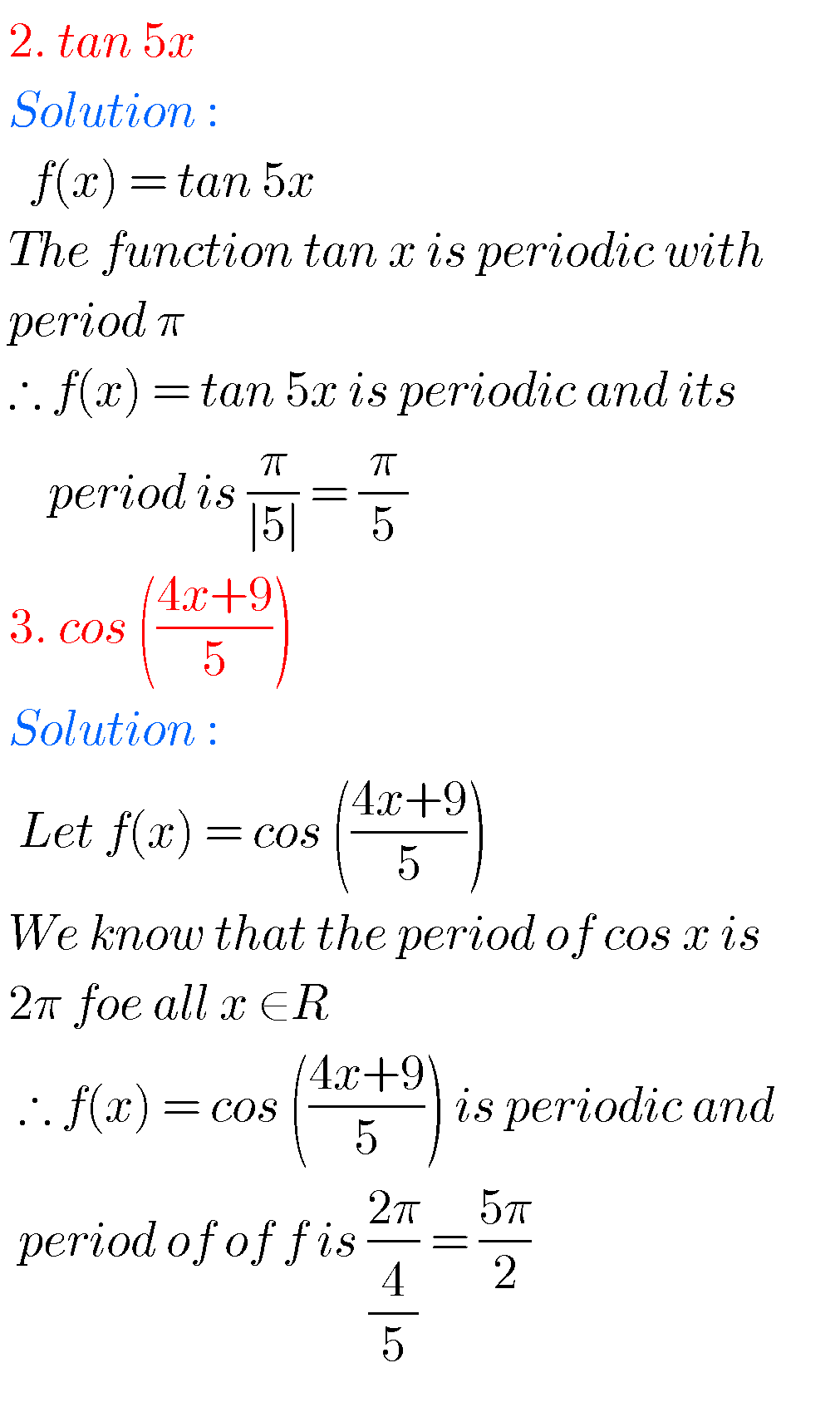## Inter maths solutions for exercise 6(b) first year,Trigonometric Ratios upto Transformations Solutions for 6(b) IA

Inter solution forMaths 1A exercise 6(b) first year Intermediate first year mathematics solutions for trigonometric ratios upto transformations exercise 6(b) are given. The solutions are very easy to understand.First you study text book lesson very well. Observe the example problems and solutions. Try them. Observe the given solutions and try them in your own method. …